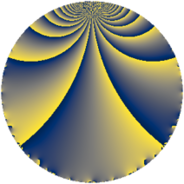Properties

 Label 130.2.bLevel $130$ Weight $2$ Character orbit 130.b Rep. character $\chi_{130}(79,\cdot)$ Character field $\Q$ Dimension $6$ Newform subspaces $1$ Sturm bound $42$ Trace bound $0$

Related objects

Defining parameters

 Level: $$N$$ $$=$$ $$130 = 2 \cdot 5 \cdot 13$$ Weight: $$k$$ $$=$$ $$2$$ Character orbit: $$[\chi]$$ $$=$$ 130.b (of order $$2$$ and degree $$1$$) Character conductor: $$\operatorname{cond}(\chi)$$ $$=$$ $$5$$ Character field: $$\Q$$ Newform subspaces: $$1$$ Sturm bound: $$42$$ Trace bound: $$0$$

Dimensions

The following table gives the dimensions of various subspaces of $$M_{2}(130, [\chi])$$.

Total New Old
Modular forms 26 6 20
Cusp forms 18 6 12
Eisenstein series 8 0 8

Trace form

 $$6 q - 6 q^{4} - 10 q^{9} + O(q^{10})$$ $$6 q - 6 q^{4} - 10 q^{9} - 4 q^{10} + 12 q^{11} + 4 q^{14} - 16 q^{15} + 6 q^{16} - 4 q^{19} + 24 q^{21} - 16 q^{25} + 6 q^{26} + 4 q^{29} - 14 q^{30} + 24 q^{31} - 8 q^{34} - 6 q^{35} + 10 q^{36} + 4 q^{40} + 4 q^{41} - 12 q^{44} - 12 q^{45} + 12 q^{46} - 26 q^{49} - 16 q^{50} - 20 q^{51} + 24 q^{54} - 4 q^{56} - 4 q^{59} + 16 q^{60} + 20 q^{61} - 6 q^{64} + 4 q^{65} + 56 q^{69} + 12 q^{70} - 16 q^{71} + 16 q^{74} - 10 q^{75} + 4 q^{76} - 56 q^{79} - 2 q^{81} - 24 q^{84} + 28 q^{85} - 24 q^{86} + 4 q^{89} - 2 q^{90} - 4 q^{91} + 20 q^{94} - 4 q^{95} - 20 q^{99} + O(q^{100})$$

Decomposition of $$S_{2}^{\mathrm{new}}(130, [\chi])$$ into newform subspaces

Label Dim. $$A$$ Field CM Traces $q$-expansion
$a_{2}$ $a_{3}$ $a_{5}$ $a_{7}$
130.2.b.a $6$ $1.038$ 6.0.3534400.1 None $$0$$ $$0$$ $$0$$ $$0$$ $$q+\beta _{5}q^{2}+(\beta _{3}-\beta _{4})q^{3}-q^{4}+(\beta _{3}+\beta _{5})q^{5}+\cdots$$

Decomposition of $$S_{2}^{\mathrm{old}}(130, [\chi])$$ into lower level spaces

$$S_{2}^{\mathrm{old}}(130, [\chi]) \cong$$ $$S_{2}^{\mathrm{new}}(65, [\chi])$$$$^{\oplus 2}$$